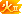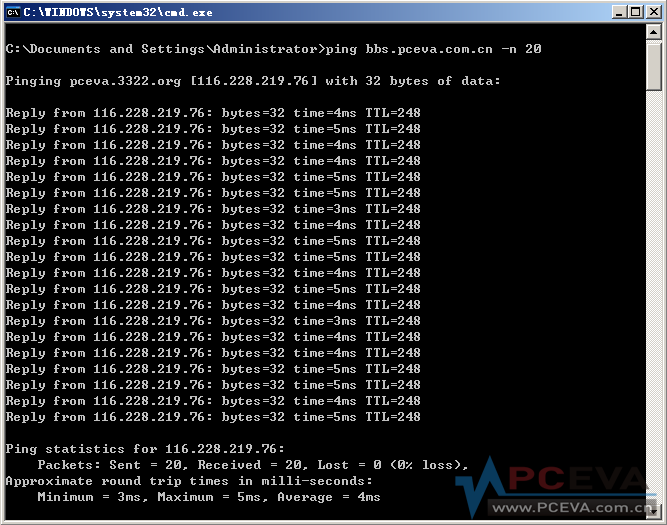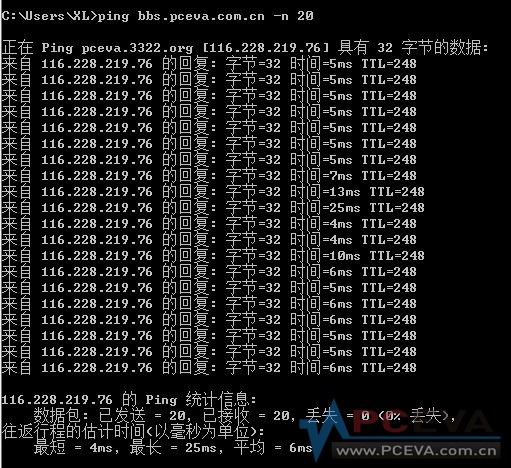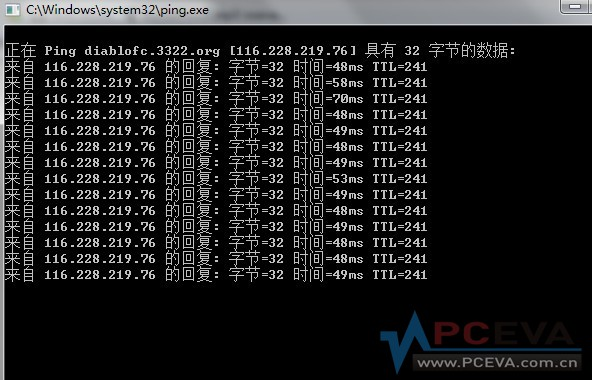# 趁着联通骨干网故障，帮忙做个测试~~~贴出结果的都有分哦[复制链接]
 じ☆ve寶寶 发表于 2012-7-11 19:34回家一看 10M联通 有点郁闷 已经比我公司的这好多了
 顺便安慰一下  大菠萝ach9999 发表于 2012-7-12 10:34顺便安慰一下  大菠萝菠萝确实劳苦功高啊~~~
 正在 Ping 116.228.219.76 具有 32 字节的数据: 来自 116.228.219.76 的回复: 字节=32 时间=39ms TTL=246 来自 116.228.219.76 的回复: 字节=32 时间=36ms TTL=246 来自 116.228.219.76 的回复: 字节=32 时间=36ms TTL=246 来自 116.228.219.76 的回复: 字节=32 时间=36ms TTL=246 来自 116.228.219.76 的回复: 字节=32 时间=39ms TTL=246 来自 116.228.219.76 的回复: 字节=32 时间=38ms TTL=246 来自 116.228.219.76 的回复: 字节=32 时间=38ms TTL=246 来自 116.228.219.76 的回复: 字节=32 时间=38ms TTL=246 来自 116.228.219.76 的回复: 字节=32 时间=35ms TTL=246 来自 116.228.219.76 的回复: 字节=32 时间=33ms TTL=246 来自 116.228.219.76 的回复: 字节=32 时间=38ms TTL=246 来自 116.228.219.76 的回复: 字节=32 时间=37ms TTL=246 来自 116.228.219.76 的回复: 字节=32 时间=36ms TTL=246 来自 116.228.219.76 的回复: 字节=32 时间=35ms TTL=246 来自 116.228.219.76 的回复: 字节=32 时间=38ms TTL=246 来自 116.228.219.76 的回复: 字节=32 时间=38ms TTL=246 来自 116.228.219.76 的回复: 字节=32 时间=38ms TTL=246 来自 116.228.219.76 的回复: 字节=32 时间=36ms TTL=246 来自 116.228.219.76 的回复: 字节=32 时间=36ms TTL=246 来自 116.228.219.76 的回复: 字节=32 时间=35ms TTL=246 116.228.219.76 的 Ping 统计信息:     数据包: 已发送 = 20，已接收 = 20，丢失 = 0 (0% 丢失)， 往返行程的估计时间(以毫秒为单位):     最短 = 33ms，最长 = 39ms，平均 = 36ms 不开BT就很正常了，唉，老房子，不知何年何月才有机会换光纤，杯具的AD啊。

### 评分

 C:\Users\Administrator>ping bbs.pceva.com.cn -n 20 正在 Ping diablofc.3322.org [116.228.219.76] 具有 32 字节的数据: 来自 116.228.219.76 的回复: 字节=32 时间=385ms TTL=242 来自 116.228.219.76 的回复: 字节=32 时间=382ms TTL=242 来自 116.228.219.76 的回复: 字节=32 时间=383ms TTL=242 来自 116.228.219.76 的回复: 字节=32 时间=411ms TTL=242 来自 116.228.219.76 的回复: 字节=32 时间=383ms TTL=242 来自 116.228.219.76 的回复: 字节=32 时间=384ms TTL=242 来自 116.228.219.76 的回复: 字节=32 时间=386ms TTL=242 来自 116.228.219.76 的回复: 字节=32 时间=374ms TTL=242 来自 116.228.219.76 的回复: 字节=32 时间=387ms TTL=242 来自 116.228.219.76 的回复: 字节=32 时间=378ms TTL=242 来自 116.228.219.76 的回复: 字节=32 时间=389ms TTL=242 来自 116.228.219.76 的回复: 字节=32 时间=378ms TTL=242 来自 116.228.219.76 的回复: 字节=32 时间=387ms TTL=242 来自 116.228.219.76 的回复: 字节=32 时间=381ms TTL=242 来自 116.228.219.76 的回复: 字节=32 时间=392ms TTL=242 来自 116.228.219.76 的回复: 字节=32 时间=382ms TTL=242 来自 116.228.219.76 的回复: 字节=32 时间=389ms TTL=242 来自 116.228.219.76 的回复: 字节=32 时间=373ms TTL=242 来自 116.228.219.76 的回复: 字节=32 时间=378ms TTL=242 来自 116.228.219.76 的回复: 字节=32 时间=380ms TTL=242 116.228.219.76 的 Ping 统计信息:     数据包: 已发送 = 20，已接收 = 20，丢失 = 0 (0% 丢失)， 往返行程的估计时间(以毫秒为单位):     最短 = 373ms，最长 = 411ms，平均 = 384ms 翻墙之后... 美国holy PV 173.224.213.11

### 评分

 C:\Users\Administrator>ping bbs.pceva.com.cn -n 20 正在 Ping diablofc.3322.org [116.228.219.76] 具有 32 字节的数据: 来自 116.228.219.76 的回复: 字节=32 时间=813ms TTL=241 来自 116.228.219.76 的回复: 字节=32 时间=776ms TTL=241 来自 116.228.219.76 的回复: 字节=32 时间=799ms TTL=241 来自 116.228.219.76 的回复: 字节=32 时间=794ms TTL=241 来自 116.228.219.76 的回复: 字节=32 时间=771ms TTL=241 来自 116.228.219.76 的回复: 字节=32 时间=810ms TTL=241 来自 116.228.219.76 的回复: 字节=32 时间=781ms TTL=241 来自 116.228.219.76 的回复: 字节=32 时间=809ms TTL=241 来自 116.228.219.76 的回复: 字节=32 时间=788ms TTL=241 来自 116.228.219.76 的回复: 字节=32 时间=811ms TTL=241 来自 116.228.219.76 的回复: 字节=32 时间=793ms TTL=241 来自 116.228.219.76 的回复: 字节=32 时间=796ms TTL=241 来自 116.228.219.76 的回复: 字节=32 时间=786ms TTL=241 来自 116.228.219.76 的回复: 字节=32 时间=786ms TTL=241 来自 116.228.219.76 的回复: 字节=32 时间=806ms TTL=241 来自 116.228.219.76 的回复: 字节=32 时间=783ms TTL=241 来自 116.228.219.76 的回复: 字节=32 时间=814ms TTL=241 来自 116.228.219.76 的回复: 字节=32 时间=786ms TTL=241 来自 116.228.219.76 的回复: 字节=32 时间=801ms TTL=241 来自 116.228.219.76 的回复: 字节=32 时间=806ms TTL=241 116.228.219.76 的 Ping 统计信息:     数据包: 已发送 = 20，已接收 = 20，丢失 = 0 (0% 丢失)， 往返行程的估计时间(以毫秒为单位):     最短 = 771ms，最长 = 814ms，平均 = 795ms 英国chestnut TH 109.169.76.163

### 评分

 公司宽带  上海电信 10M光纤基本秒开 赛扬单核2.66加768M内存  权贵大图无爱.....

### 评分

 上海电信  2M ，校园网。。。### 评分

 苏州电信 4M小水管 Microsoft Windows [版本 6.1.7601] 版权所有 (c) 2009 Microsoft Corporation。保留所有权利。 C:\Users\Lotus>ping bbs.pceva.com.cn -n 20 正在 Ping diablofc.3322.org [116.228.219.76] 具有 32 字节的数据: 来自 116.228.219.76 的回复: 字节=32 时间=500ms TTL=241 来自 116.228.219.76 的回复: 字节=32 时间=494ms TTL=241 来自 116.228.219.76 的回复: 字节=32 时间=495ms TTL=241 来自 116.228.219.76 的回复: 字节=32 时间=501ms TTL=241 来自 116.228.219.76 的回复: 字节=32 时间=493ms TTL=241 来自 116.228.219.76 的回复: 字节=32 时间=495ms TTL=241 来自 116.228.219.76 的回复: 字节=32 时间=502ms TTL=241 来自 116.228.219.76 的回复: 字节=32 时间=493ms TTL=241 来自 116.228.219.76 的回复: 字节=32 时间=508ms TTL=241 来自 116.228.219.76 的回复: 字节=32 时间=496ms TTL=241 来自 116.228.219.76 的回复: 字节=32 时间=512ms TTL=241 来自 116.228.219.76 的回复: 字节=32 时间=521ms TTL=241 来自 116.228.219.76 的回复: 字节=32 时间=515ms TTL=241 来自 116.228.219.76 的回复: 字节=32 时间=516ms TTL=241 来自 116.228.219.76 的回复: 字节=32 时间=519ms TTL=241 来自 116.228.219.76 的回复: 字节=32 时间=523ms TTL=241 来自 116.228.219.76 的回复: 字节=32 时间=526ms TTL=241 来自 116.228.219.76 的回复: 字节=32 时间=526ms TTL=241 来自 116.228.219.76 的回复: 字节=32 时间=523ms TTL=241 来自 116.228.219.76 的回复: 字节=32 时间=530ms TTL=241 116.228.219.76 的 Ping 统计信息:     数据包: 已发送 = 20，已接收 = 20，丢失 = 0 (0% 丢失)， 往返行程的估计时间(以毫秒为单位):     最短 = 493ms，最长 = 530ms，平均 = 509ms C:\Users\Lotus>ping bbs.pceva.com.cn -n 20 正在 Ping pceva.3322.org [116.228.219.76] 具有 32 字节的数据: 来自 116.228.219.76 的回复: 字节=32 时间=19ms TTL=247 来自 116.228.219.76 的回复: 字节=32 时间=20ms TTL=247 来自 116.228.219.76 的回复: 字节=32 时间=20ms TTL=247 来自 116.228.219.76 的回复: 字节=32 时间=19ms TTL=247 来自 116.228.219.76 的回复: 字节=32 时间=21ms TTL=247 来自 116.228.219.76 的回复: 字节=32 时间=20ms TTL=247 来自 116.228.219.76 的回复: 字节=32 时间=19ms TTL=247 来自 116.228.219.76 的回复: 字节=32 时间=20ms TTL=247 来自 116.228.219.76 的回复: 字节=32 时间=18ms TTL=247 来自 116.228.219.76 的回复: 字节=32 时间=20ms TTL=247 来自 116.228.219.76 的回复: 字节=32 时间=19ms TTL=247 来自 116.228.219.76 的回复: 字节=32 时间=19ms TTL=247 来自 116.228.219.76 的回复: 字节=32 时间=20ms TTL=247 来自 116.228.219.76 的回复: 字节=32 时间=20ms TTL=247 来自 116.228.219.76 的回复: 字节=32 时间=19ms TTL=247 来自 116.228.219.76 的回复: 字节=32 时间=21ms TTL=247 来自 116.228.219.76 的回复: 字节=32 时间=19ms TTL=247 来自 116.228.219.76 的回复: 字节=32 时间=20ms TTL=247 来自 116.228.219.76 的回复: 字节=32 时间=20ms TTL=247 来自 116.228.219.76 的回复: 字节=32 时间=19ms TTL=247 116.228.219.76 的 Ping 统计信息:     数据包: 已发送 = 20，已接收 = 20，丢失 = 0 (0% 丢失)， 往返行程的估计时间(以毫秒为单位):     最短 = 18ms，最长 = 21ms，平均 = 19ms 第一个是开了代理的，后一个是关了的。但是关了不能访问。万恶的绿坝娘。

### 评分

 C:\Users\XXXXXX>ping bbs.pceva.com.cn -n 20 正在 Ping diablofc.3322.org [116.228.219.76] 具有 32 字节的数据: 来自 116.228.219.76 的回复: 字节=32 时间=438ms TTL=229 来自 116.228.219.76 的回复: 字节=32 时间=439ms TTL=229 来自 116.228.219.76 的回复: 字节=32 时间=438ms TTL=229 来自 116.228.219.76 的回复: 字节=32 时间=480ms TTL=229 来自 116.228.219.76 的回复: 字节=32 时间=438ms TTL=229 来自 116.228.219.76 的回复: 字节=32 时间=438ms TTL=229 来自 116.228.219.76 的回复: 字节=32 时间=438ms TTL=229 来自 116.228.219.76 的回复: 字节=32 时间=442ms TTL=229 来自 116.228.219.76 的回复: 字节=32 时间=442ms TTL=229 来自 116.228.219.76 的回复: 字节=32 时间=445ms TTL=229 来自 116.228.219.76 的回复: 字节=32 时间=438ms TTL=229 来自 116.228.219.76 的回复: 字节=32 时间=439ms TTL=229 来自 116.228.219.76 的回复: 字节=32 时间=441ms TTL=229 来自 116.228.219.76 的回复: 字节=32 时间=439ms TTL=229 来自 116.228.219.76 的回复: 字节=32 时间=441ms TTL=229 来自 116.228.219.76 的回复: 字节=32 时间=439ms TTL=229 来自 116.228.219.76 的回复: 字节=32 时间=439ms TTL=229 来自 116.228.219.76 的回复: 字节=32 时间=453ms TTL=229 来自 116.228.219.76 的回复: 字节=32 时间=437ms TTL=229 来自 116.228.219.76 的回复: 字节=32 时间=438ms TTL=229 116.228.219.76 的 Ping 统计信息:     数据包: 已发送 = 20，已接收 = 20，丢失 = 0 (0% 丢失)， 往返行程的估计时间(以毫秒为单位):     最短 = 437ms，最长 = 480ms，平均 = 442ms from EU

### 评分

 最近几天登陆网站超级超级慢啊，有啥解决的办法没有。 联通3M。 正在 Ping diablofc.3322.org [116.228.219.76] 具有 32 字节的数据: 请求超时。 请求超时。 来自 116.228.219.76 的回复: 字节=32 时间=250ms TTL=243 来自 116.228.219.76 的回复: 字节=32 时间=250ms TTL=243 来自 116.228.219.76 的回复: 字节=32 时间=254ms TTL=243 请求超时。 请求超时。 请求超时。 请求超时。 来自 116.228.219.76 的回复: 字节=32 时间=251ms TTL=243 请求超时。 请求超时。 来自 116.228.219.76 的回复: 字节=32 时间=249ms TTL=243 请求超时。 请求超时。 请求超时。 来自 116.228.219.76 的回复: 字节=32 时间=252ms TTL=243 来自 116.228.219.76 的回复: 字节=32 时间=251ms TTL=243 来自 116.228.219.76 的回复: 字节=32 时间=253ms TTL=243 请求超时。 116.228.219.76 的 Ping 统计信息:     数据包: 已发送 = 20，已接收 = 8，丢失 = 12 (60% 丢失)， 往返行程的估计时间(以毫秒为单位):     最短 = 249ms，最长 = 254ms，平均 = 251ms

### 评分

 一直不知道上海竟然还有联通

### 点评

 还有吗？继续放分哦~~~~沈阳 6M 铁通  估计也是租的联通线路

### 评分gmx168 + 10 不是联通啦，肯定是电信的线路。.
 北京联通，小区LAN，实际3M 正在 Ping diablofc.3322.org [116.228.219.76] 具有 32 字节的数据: 来自 116.228.219.76 的回复: 字节=32 时间=206ms TTL=243 来自 116.228.219.76 的回复: 字节=32 时间=206ms TTL=243 来自 116.228.219.76 的回复: 字节=32 时间=205ms TTL=243 来自 116.228.219.76 的回复: 字节=32 时间=204ms TTL=243 来自 116.228.219.76 的回复: 字节=32 时间=206ms TTL=243 来自 116.228.219.76 的回复: 字节=32 时间=206ms TTL=243 来自 116.228.219.76 的回复: 字节=32 时间=205ms TTL=243 来自 116.228.219.76 的回复: 字节=32 时间=206ms TTL=243 来自 116.228.219.76 的回复: 字节=32 时间=207ms TTL=243 来自 116.228.219.76 的回复: 字节=32 时间=205ms TTL=243 来自 116.228.219.76 的回复: 字节=32 时间=204ms TTL=243 来自 116.228.219.76 的回复: 字节=32 时间=204ms TTL=243 来自 116.228.219.76 的回复: 字节=32 时间=205ms TTL=243 来自 116.228.219.76 的回复: 字节=32 时间=206ms TTL=243 来自 116.228.219.76 的回复: 字节=32 时间=206ms TTL=243 来自 116.228.219.76 的回复: 字节=32 时间=206ms TTL=243 来自 116.228.219.76 的回复: 字节=32 时间=205ms TTL=243 来自 116.228.219.76 的回复: 字节=32 时间=205ms TTL=243 来自 116.228.219.76 的回复: 字节=32 时间=206ms TTL=243 来自 116.228.219.76 的回复: 字节=32 时间=204ms TTL=243 116.228.219.76 的 Ping 统计信息:     数据包: 已发送 = 20，已接收 = 20，丢失 = 0 (0% 丢失)， 往返行程的估计时间(以毫秒为单位):     最短 = 204ms，最长 = 207ms，平均 = 205ms

### 评分

 tracert了一下 通过最多 30 个跃点跟踪 到 diablofc.3322.org [116.228.219.76] 的路由:   1    <1 毫秒   <1 毫秒   <1 毫秒 NTSD [192.168.1.1]   2     *        *        *     请求超时。   3     4 ms    <1 毫秒   <1 毫秒 61.148.174.33   4     1 ms     1 ms     1 ms  124.65.58.253   5     1 ms     1 ms     1 ms  61.148.153.45   6     1 ms     1 ms     1 ms  123.126.0.85   7     1 ms     1 ms     1 ms  219.158.5.66   8   200 ms   198 ms   200 ms  219.158.35.98   9     *      263 ms   210 ms  202.97.46.61 10   208 ms   204 ms   200 ms  202.101.63.129 11     *      200 ms   226 ms  61.152.81.22 12   209 ms   209 ms   209 ms  124.74.209.202 13   205 ms   204 ms   204 ms  124.74.50.134 14   209 ms   209 ms   210 ms  124.74.67.198 15   203 ms   202 ms   205 ms  116.228.219.76 跟踪完成。 感觉是从哪儿绕了一圈又绕回来了……这几天访问论坛真不是一般的卡
 nighttob 发表于 2012-7-13 14:50tracert了一下 通过最多 30 个跃点跟踪 1    <1 毫秒   <1 毫秒   <1 毫秒 NTSD [192.168.1.1]   2     *        *        *     请求超时。   3     4 ms    <1 毫秒   <1 毫秒 61.148.174.33   4     1 ms     1 ms     1 ms  124.65.58.253   5     1 ms     1 ms     1 ms  61.148.153.45   6     1 ms     1 ms     1 ms  123.126.0.85   7     1 ms     1 ms     1 ms  219.158.5.66   8   200 ms   198 ms   200 ms  219.158.35.98          北京市 联通   9     *      263 ms   210 ms  202.97.46.61                  中国 电信骨干网 10   208 ms   204 ms   200 ms  202.101.63.129 11     *      200 ms   226 ms  61.152.81.22 12   209 ms   209 ms   209 ms  124.74.209.202 13   205 ms   204 ms   204 ms  124.74.50.134 14   209 ms   209 ms   210 ms  124.74.67.198 15   203 ms   202 ms   205 ms  116.228.219.76
 看了一下，电信线路的帖子多，联通的少~~~继续贴吧，我继续加分
 您需要登录后才可以回帖 登录 | 注册 本版积分规则 回帖并转播 回帖后跳转到最后一页